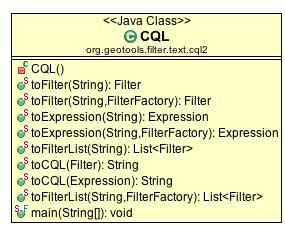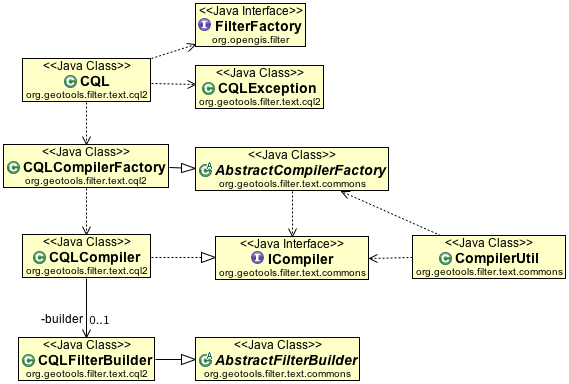# Internal¶

This package is dedicated to support the OGC Common Query Language, version 2.0.1, as a query predicate language inside GeoTools. The rest of this document describe the syntax rules and the parser design.

## CQL Interface¶

This diagram presents the package interface. In parser’s protocol methods performs the parsing of CQL and builds the filter.## CQL Implementation¶

The figure shows the principal class in the parser and build process. CQLParser does a top down analysis of the input string and makes the parsing tree. Each time CQLParser builds a node, it makes a call to CQLCompiler, that implements the semantic actions related and builds the product or sub product required to make the Filter at the end of the parsing process## BNF¶

The BNF is reproduced bellow to indicate the extensions made, which are highlighted in bold characters. The original BNF was extended thinking in SQL where expression. Moreover, we found some errors in the specification and made some corrections, that will help to use and analysis the CQL language.

Syntax Rules:

```<sequence of search conditions> ::= 
<search condition>
|  <sequence of search conditions> <semicolon> <search condition>

<search condition> ::= <boolean value expression>
<boolean value expression> ::=
<boolean term>
| <boolean value expression> OR <boolean term>

<boolean term> ::=
<boolean factor>
| <boolean term> AND <boolean factor>

<boolean factor> ::= [ NOT ] <boolean primary>

<boolean primary> ::=
<predicate>
| <routine invocation>
| <routine invocation>
| "(" <search condition> ")"
<predicate> ::=
<comparison predicate>
| <text predicate>
| <null predicate>
| <temporal predicate>
| <classification predicate> 
| <existence_predicate>
| <between predicate>
| <include exclude predicate>
```

Existence Predicate:

```<existence_predicate> :=
<attribute_name> EXISTS
| <attribute_name> DOES-NOT-EXIST
```

Comparison Predicate:

```<comparison predicate> ::=
<attribute name> <comp op> <literal>
| <attribute name> <comp op> <expression>
<comp op> ::= <equals operator>
| <not equals operator>
| <less than operator>
| <greater than operator>
| <less than or equals operator>
| <greater than or equals operator>
```

Text Predicate (or Like Predicate):

```<text predicate> ::= <attribute name> [ NOT ] LIKE <character pattern>

<character pattern> ::= <character string literal>

(* pattern examples :
attribute like '%contains_this%'
attribute like 'begins_with_this%'
attribute like '%ends_with_this'
attribute like 'd_ve' will match 'dave' or 'dove'
attribute not like '%will_not_contain_this%'
attribute not like 'will_not_begin_with_this%'
attribute not like '%will_not_end_with_this' *)
```

NULL Predicate:

```<null predicate> ::= <attribute name> IS [ NOT ] NULL
```

Between Predicate:

```<between predicate> ::= <attribute name> [ NOT ] BETWEEN <literal> AND < literal > 
```

Temporal Predicate:

```<temporal predicate> ::=
<attribute_name> "BEFORE" <date-time expression>
| <attribute_name> "BEFORE OR DURING" <period>
| <attribute_name> "DURING" <period>
| <attribute_name> "DURING OR AFTER" <period>
| <attribute_name> "AFTER" <date-time expression>

<date-time expression ::= <date-time> | <period>

<date-time> ::= <full-date> "T" <UTC-time>
<full_date> ::= <date-year> "-" <date-month> "-" <date-day>
<date-year> ::= <digit><digit><digit><digit>
<date-month> ::= <digit><digit>
<date-day> ::= <digit><digit>
<UTC-time> ::=<time-hour>":"<time-minute>":"<time-second> [<time-zone-offset>]
<time-zone-offset> ::= "Z" | <sign> <time-hour>":"<time-minute>

<time-hour> ::= <digit><digit>
<time-minute> ::= <digit><digit>
<time-second> ::= <digit><digit>[.<digit>...]
<duration> ::= "P" <dur-date> | "T"<dur-time>
<dur-date> ::= <dur-day> | <dur-month> | <dur-year> [<dur-time>]
<dur-day> ::= <digit>... "D"
<dur-month> ::= <digit>... "M" [<dur-day>]
<dur-year> ::= <didit>... "Y" [<dur-month>]
<dur-time> ::= <dur-hour> | <dur-minute> | <dur-second>
<dur-hour> ::= <digit>... "H" [<dur-minute>]
<dur-minute> ::= <digit>... "M" [<dur-second>]
<dur-second> ::= <digit>... "S"
<period> ::=
<date-time> / <date-time>
| <date-time> / <duration>
| <duration> / <date-time>
```

INCLUDE/EXCLUDE Predicate:

```<include exclude predicate> ::= "INCLUDE" | "EXCLUDE"
```

Expression:

```<expression> ::= <term> { <addition operator>  <term> }
<addition operator>::= <plus sign> | <minus sign>

<term> ::=  <factor> { <multiplication operator> <factor> }
<multiplication operator> ::= <asterisk> | <solidus>

<factor> ::=
<function>
| <literal>
| <attribute>
| ( <expression> )
| [ <expression> ]

<function> ::= <identifier> "(" ( <function arguments> ("," <function arguments> )* )? ")"

<function arguments> ::= <literal> | <attribute> | <expression>
<literal> ::= <signed numeric literal>| <general literal>

<signed numeric literal> ::= [ <sign> ] <unsigned numeric literal>

<general literal> ::=
<character string literal>
| <boolean literal>
| <geography literal

<boolean literal> ::= "TRUE" | "FALSE" | "UNKNOWN"
```

Geo-Routine and Relational Geo-Operations:

```<routine invocation> ::=
<geoop name><georoutine argument list>
| <relgeoop name><relgeoop argument list>
| <bbox geoop>
| <relate geop>

<routine name> ::= < attribute name>

<geoop name> ::= "EQUALS" | "DISJOINT" | "INTERSECTS" | "TOUCHES" | "CROSSES" | "WITHIN" | "CONTAINS" | "OVERLAPS"

<bbox geoop>::= "BBOX" "(" <attribute> ","<min X> ","<min Y> ","<max X> ","<max Y>["," <crs>] ")"  

<min X> ::= <signed numerical literal>
<min Y> ::= <signed numerical literal>
<max X> ::= <signed numerical literal>
<max Y> ::= <signed numerical literal>
<crs> ::=  ... (* default: EPSG:4326. *)

<relate geop> ::= "RELATE" "(" <attribute name>"," <geometry literal>"," <DE-9IM pattern> ")"

<DE-9IM pattern> ::= <dimension simbol><dimension simbol><dimension simbol><dimension simbol><dimension simbol><dimension simbol><dimension simbol><dimension simbol><dimension simbol>
<dimension simbol> ::= "*"| "T" | "F" | "0" | "1" | "2"> |

<relgeoop name> ::= "DWITHIN" | "BEYOND"

<argument list> ::= "(" [ <positional arguments> ] ")"

<positional arguments> ::= <argument> [ { "," <argument> } ...]

<argument> ::= <literal> | <attribute name>

<georoutine argument list> ::=  "("<attribute name>","<geometry literal>")"

<relgeoop argument list> ::= "("<attribute name>","<geometry literal>","<tolerance>")"

<tolerance> ::=<unsigned numeric literal>","<distance units>

<distance units> ::= = "feet" | "meters" | "statute miles" | "nautical miles" | "kilometers"
```

Geometry Literal:

```<geometry literal> :=
<Point Tagged Text>
| <LineString Tagged Text>
| <Polygon Tagged Text>
| <MultiPoint Tagged Text>
| <MultiLineString Tagged Text>
| <MultiPolygon Tagged Text>
| <GeometryCollection Tagged Text>
| <Envelope Tagged Text>

<Point Tagged Text> ::= POINT <Point Text>

<LineString Tagged Text> ::= LINESTRING <LineString Text>

<Polygon Tagged Text> ::= POLYGON <Polygon Text>

<MultiPoint Tagged Text> ::= MULTIPOINT <Multipoint Text>

<MultiLineString Tagged Text> ::= MULTILINESTRING <MultiLineString Text>

<MultiPolygon Tagged Text> ::= MULTIPOLYGON <MultiPolygon Text>

<GeometryCollection Tagged Text> ::=GEOMETRYCOLLECTION <GeometryCollection Text>

<Point Text> := EMPTY | "(" <Point> ")"
<Point> := <x><space><y>
<x> := numeric literal
<y> := numeric literal
<LineString Text> := EMPTY | "(" <Point> \{","<Point >\}...")"
<Polygon Text> := EMPTY | "("<LineString Text>\{","<LineString Text> \}...")"
<Multipoint Text> := EMPTY | "("<Point Text>\{","<Point Text >\}...")"
<MultiLineString Text> := EMPTY | "("<LineString Text>\{","<LineString Text>\}...")"
<MultiPolygon Text> := EMPTY | "("<Polygon Text>\{","<Polygon Text>\}...")"
<GeometryCollection Text> := EMPTY | "("<Geometry Tagged Text>\{","<Geometry Tagged Text>\}...")"
<Envelope Tagged Text> ::= ENVELOPE <Envelope Text>
<Envelope Text> ::= EMPTY |
"("<WestBoundLongitude>","
<EastBoundLongitude>","
<NorthBoundLatitude>","
<SouthBoundLatitude>< ")"
<WestBoundLongitude> ::= numeric literal
<EastBoundLongitude> ::= numeric literal
<NorthBoundLatitude> ::= numeric literal
<SouthBoundLatitude> ::= numeric literal
```

Lexical Rules:

```<SQL terminal character> ::= <SQL language character>
<SQL language character> ::= <simple Latin letter>
| <digit>
| <SQL special character>
<simple Latin letter> ::= <simple Latin upper case letter>
| <simple Latin lower case letter>
<simple Latin upper case letter> ::=
A | B | C | D | E | F | G | H | I | J | K | L | M | N | O
| P | Q | R | S | T | U | V | W | X | Y | Z
<simple Latin lower case letter> ::=
a | b | c | d | e | f | g | h | i | j | k | l | m | n | o
| p | q | r | s | t | u | v | w | x | y | z
<digit> ::=
0 | 1 | 2 | 3 | 4 | 5 | 6 | 7 | 8 | 9
<SQL special character> ::= <space>
| <double quote>
| <percent>
| <ampersand>
| <quote>
| <left paren>
| <right paren>
| <asterisk>
| <plus sign>
| <comma>
| <minus sign>
| <period>
| <solidus>
| <colon>
| <semicolon>
| <less than operator>
| <equals operator>
| <greater than operator>
| <question mark>
| <left bracket>
| <right bracket>
| <circumflex>
| <underscore>
| <vertical bar>
| <left brace>
| <right brace>
<space> ::= /*space character in character set in use
In ASCII it would be 40*/
<double quote> ::= "
<percent> ::= %
<ampersand> ::= &
<quote> ::= '
<left paren> ::= (
<right paren> ::= )
<asterisk> ::= *
<plus sign> ::= +
<comma> ::= ,
<minus sign> ::= -
<period> ::= .
<solidus> ::= /
<colon> ::= :
<semicolon> ::= ;
<less than operator> ::= <
<equals operator> ::= =
<greater than operator> ::= >
<question mark> ::= ?
<left bracket> ::= [
<right bracket> ::= ]
<circumflex> ::= ^
<underscore> ::= _
<vertical bar> ::= |
<left brace> ::={
<right brace> ::=}
<separator> ::= { <comment> | <space> | <newline> }...
<token> ::= <nondelimiter token>
| <delimiter token>
<nondelimiter token> ::= <regular identifier>
| <key word>
| <unsigned numeric literal>
<key word> ::= <reserved word>
<reserved word> ::= NOT | AND | OR | LIKE |
IS | NULL |
EXISTS | DOES-NOT-EXIST |
DURING | AFTER | BEFORE
INCLUDE | EXCLUDE |
TRUE | FALSE |
EQUALS | DISJOINT | INTERSECTS | TOUCHES | CROSSES | WITHIN | CONTAINS| OVERLAPS | RELATE | DWITHIN | BEYOND |
POINT | LINESTRING | POLYGON |
MULTIPOINT | MULTILINESTRING | MULTIPOLYGON | GEOMETRYCOLLECTION
```

Numeric:

```<unsigned numeric literal> ::= <exact numeric literal>
| <approximate numeric literal>
<exact numeric literal> ::=
<unsigned integer> [<period>[<unsigned integer> ] ]
| <period> <unsigned integer>
<unsigned integer> ::= <digit>...
<approximate numeric literal> ::= <mantissa> E <exponent>
<mantissa> ::= <exact numeric literal>
<exponent> ::= <signed integer>
<signed integer> ::= [ <sign> ] <unsigned integer>
<sign> ::= <plus sign> | <minus sign>
```

Delimiter:

```<delimiter token> ::= <character string literal>
| <SQL special character>
| <not equals operator>
| <greater than or equals operator>
| <less than or equals operator>
| <concatenation operator>
| <double greater than operator>
| <right arrow>
| <left bracket>
| <right bracket>
<character string literal> ::=
<quote> [ <character representation>... ] <quote>
<character representation> ::= <nonquote character> | <quote symbol>
<quote symbol> ::= <quote><quote>
<not equals operator> ::= <>
<greater than or equals operator> ::= >=
<less than or equals operator> ::= <=
```

Character String Literal:

```<character string literal> ::=
<quote> [ <character representation>... ] <quote>
<character representation> ::= <nonquote character> | <quote symbol>

<quote symbol> ::= <quote><quote>
```

Identifier

The following section is intended to give context for identifier and namespaces. It assumes that the default namespace is specified in the query request and does not allow any overrides of the namespace:

```<regular identifier> ::= <identifier body>
<identifier body> ::=
<identifier start> [ { <underscore> | <identifier part> }... ]
<identifier start> ::= <simple latin letter>
<identifier part> ::= <identifier start>
| <digit>

<identifier> ::= <identifier start> [ { <colon> | <identifier part> }... ]
<identifier start> ::= <simple Latin letter>
<identifier part> ::= <simple Latin letter> | <digit>
<attribute name> ::= <simple attribute name> | <compound attribute name>
<simple attribute name> ::= <identifier>
<compound attribute name> ::= <identifier><period>
[{<identifier><period>}...]
<simple attribute name>
```